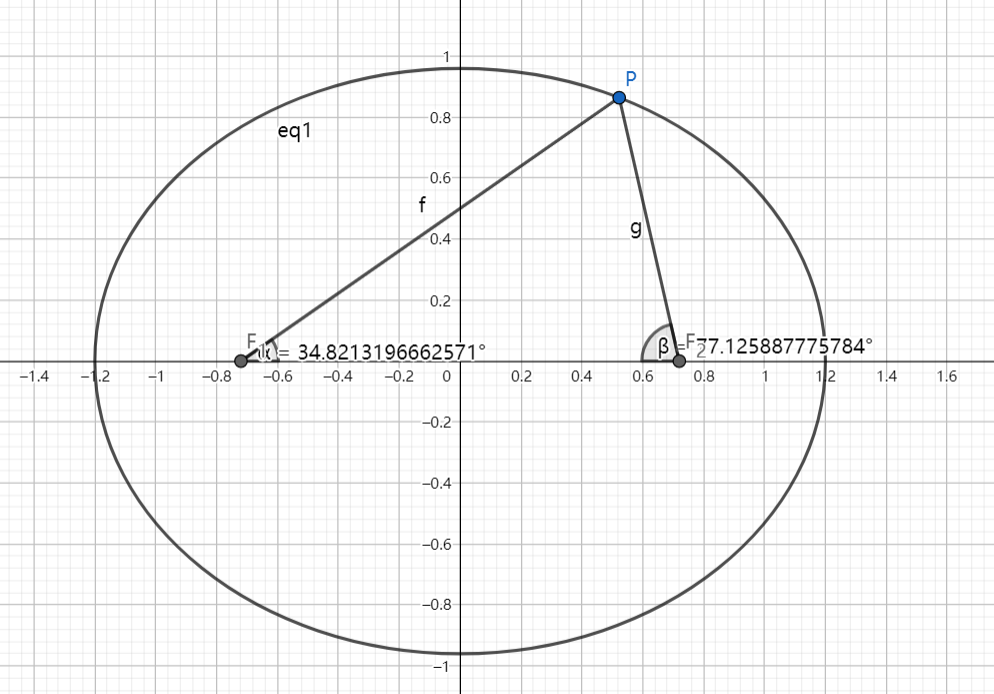# SAT1000 - P766

Geometry Level pendingAs shown above, the left and right focus of the ellipse: $\dfrac{x^2}{a^2}+\dfrac{y^2}{b^2}=1 (a>b>0)$ are $F_1(-c,0), F_2(c,0)$.

If there exists point $P$ such that $\dfrac{a}{\sin \angle PF_1F_2}=\dfrac{c}{\sin \angle PF_2F_1}$, find the range of the eccentricity of the ellipse.

The range can be expressed as $(l,r)$. Submit $\lfloor 1000(2r-l) \rfloor$.

Have a look at my problem set: SAT 1000 problems

×